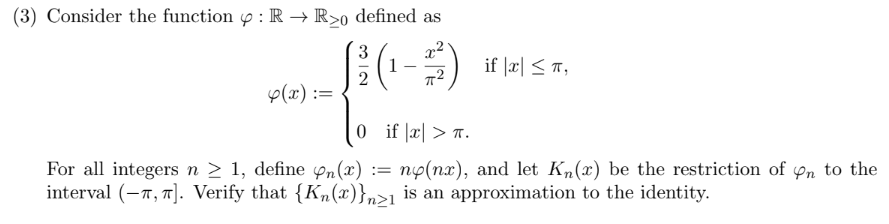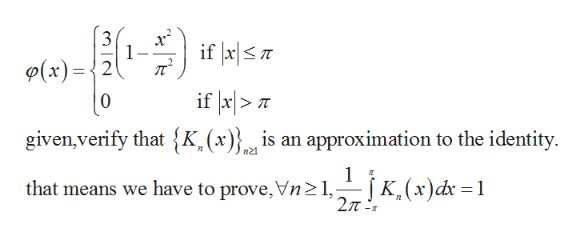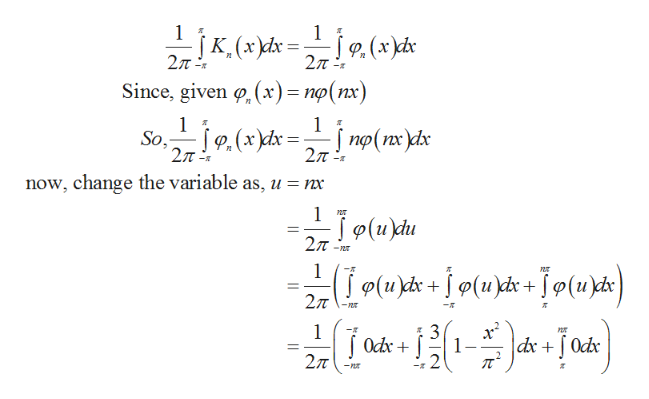# (3) Consider the functiondefined as: R -> R>0if laT12p(x)0 if a>np(nx), and let Kn(x) be the restriction of pn to theFor all integers n > 1, define pn(x) :=interval (, . Verify that {Kn(x)}n>1 is an approximation to the identity

Question
9 viewshelp_outlineImage Transcriptionclose(3) Consider the function defined as : R -> R>0 if laT 1 2 p(x) 0 if a> np(nx), and let Kn(x) be the restriction of pn to the For all integers n > 1, define pn(x) := interval (, . Verify that {Kn(x)}n>1 is an approximation to the identity fullscreen
check_circle

Step 1

Consider the given function which is defined as,help_outlineImage Transcriptioncloseif a|S 1 л" (x)=2 if x|>T given,verify that {K,(x)}is an approximation to the identity |0 n21 1 that means we have to prove,Vn21, fullscreen
Step 2

The above integral function satisf...help_outlineImage Transcriptionclose1 K, (xx 2T f9,(x)dx 2л Since, given . (х) — по(пх) 1 . So, 2л P(x)dx 27T -z now, change the variable as, u = nx 1 T Jo(изи 2л 1 (ucs+(ux +Jp(ux} 1 Odc 27T 3 1 dx0dx fullscreen

### Want to see the full answer?

See Solution

#### Want to see this answer and more?

Solutions are written by subject experts who are available 24/7. Questions are typically answered within 1 hour.*

See Solution
*Response times may vary by subject and question.
Tagged in

### Math